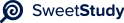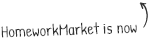•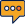0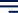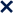1 / 21100%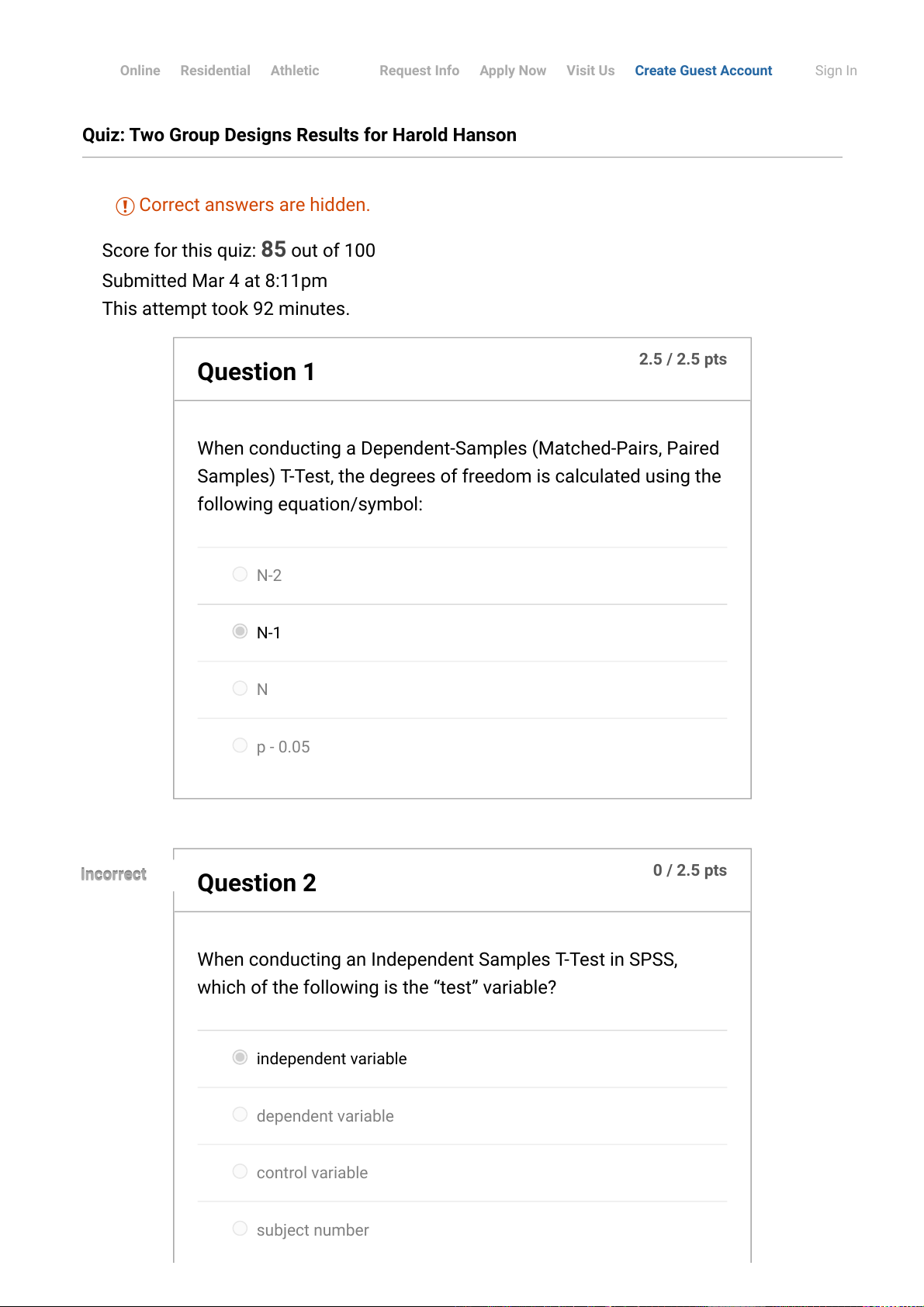Quiz: Two Group Designs Results for Harold Hanson
Score for this quiz: 85 out of 100
Submitted Mar 4 at 8:11pm
This attempt took 92 minutes.
2.5 / 2.5 pts
Question 1
When conducting a Dependent-Samples (Matched-Pairs, Paired
Samples) T-Test, the degrees of freedom is calculated using the
following equation/symbol:
N-2
N-1
N
p - 0.05
0 / 2.5 pts
Question 2
Incorrect
Incorrect
When conducting an Independent Samples T-Test in SPSS,
which of the following is the “test” variable?
independent variable
dependent variable
control variable
subject number
Online
Residential
Athletic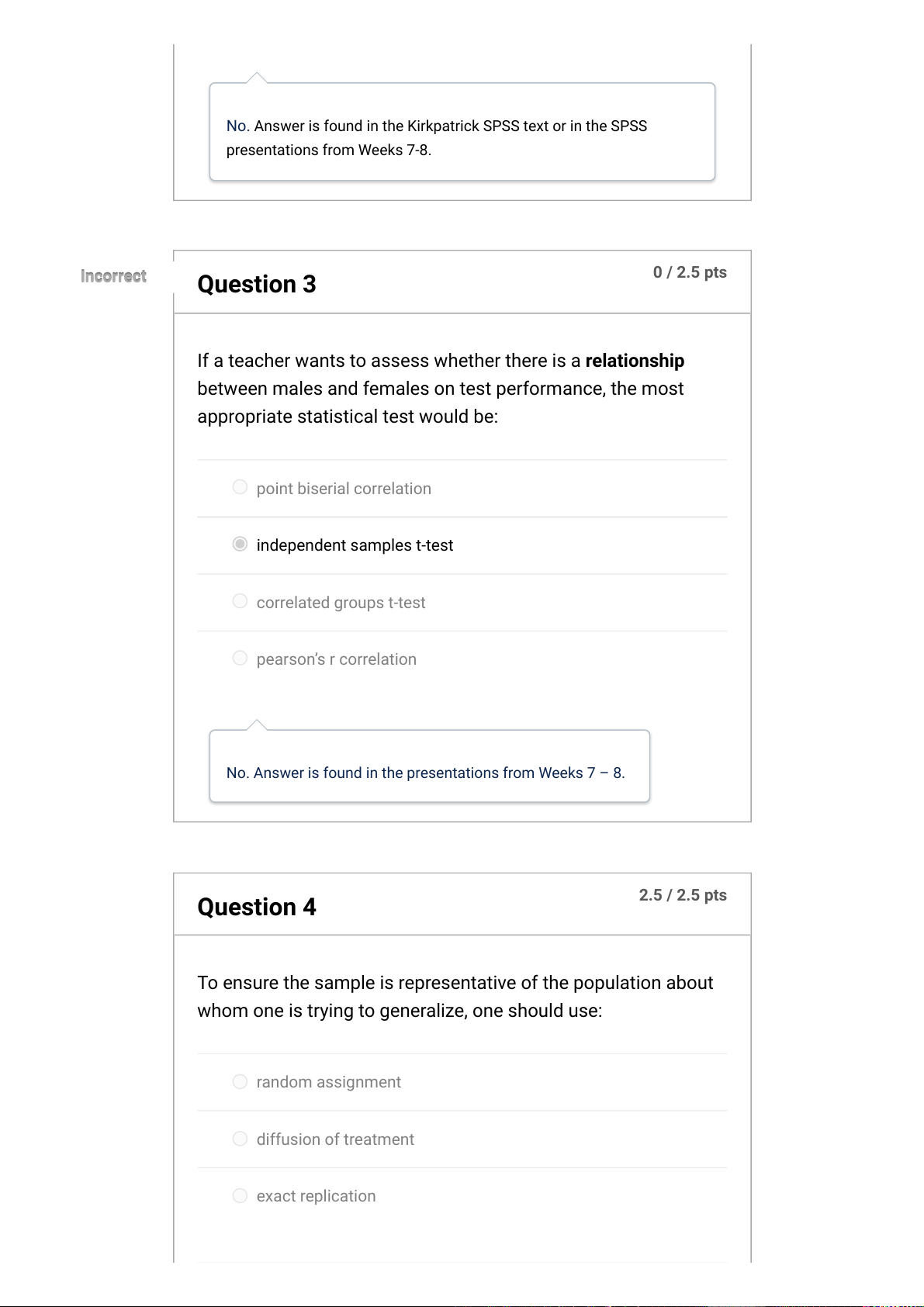No.Answer is found in the Kirkpatrick SPSS text or in the SPSS
presentations from Weeks 7-8.
0 / 2.5 pts
Question 3
Incorrect
Incorrect
If a teacher wants to assess whether there is a relationship
between males and females on test performance, the most
appropriate statistical test would be:
point biserial correlation
independent samples t-test
correlated groups t-test
pearsons r correlation
No. Answer is found in the presentations from Weeks 7 8.
2.5 / 2.5 pts
Question 4
To ensure the sample is representative of the population about
whom one is trying to generalize, one should use:
random assignment
diffusion of treatment
exact replication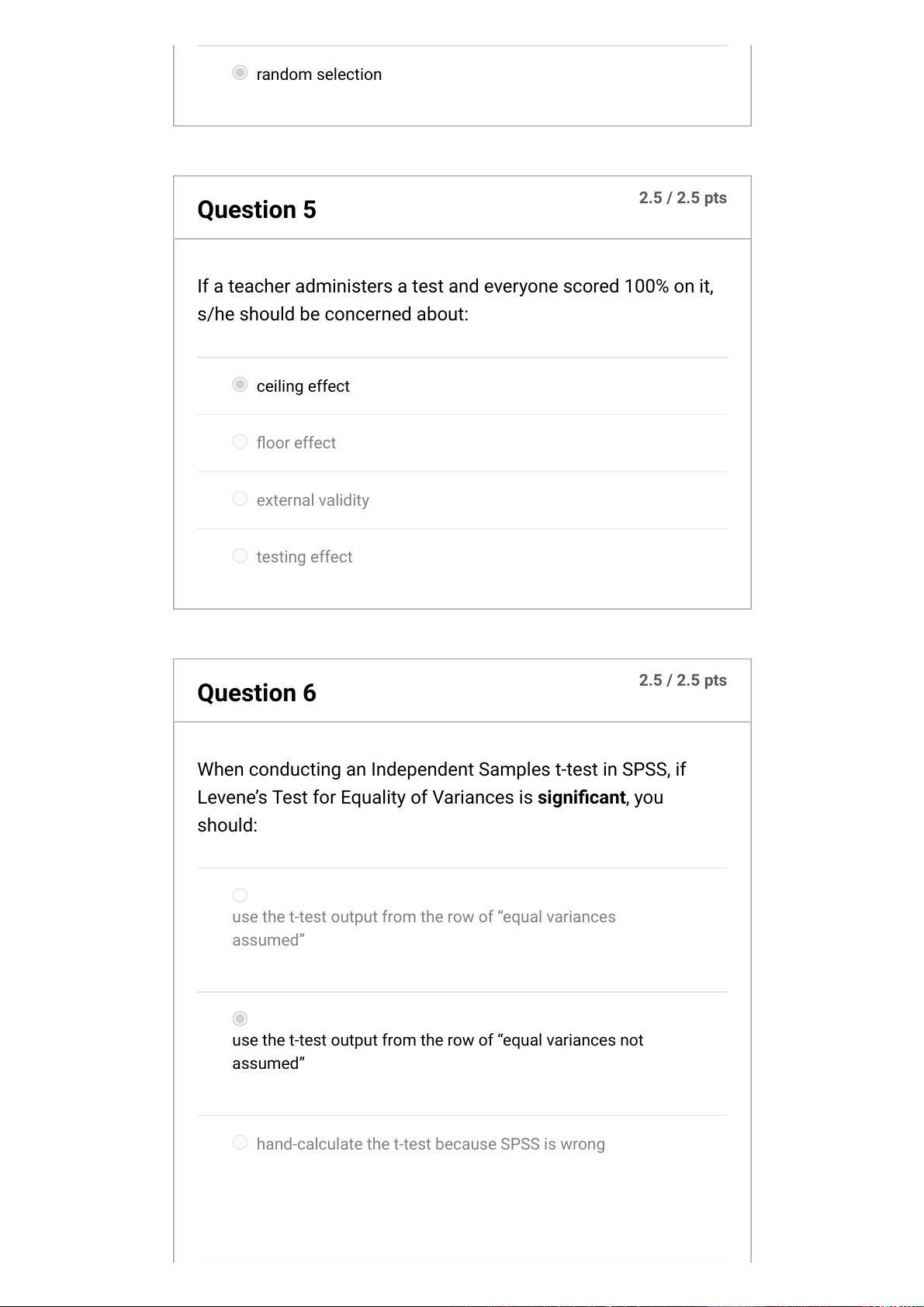random selection
2.5 / 2.5 pts
Question 5
If a teacher administers a test and everyone scored 100% on it,
ceiling effect
oor effect
external validity
testing effect
2.5 / 2.5 pts
Question 6
When conducting an Independent Samples t-test in SPSS, if
Levenes Test for Equality of Variances is signicant, you
should:
use the t-test output from the row of equal variances
assumed”
use the t-test output from the row of equal variances not
assumed”
hand-calculate the t-test because SPSS is wrong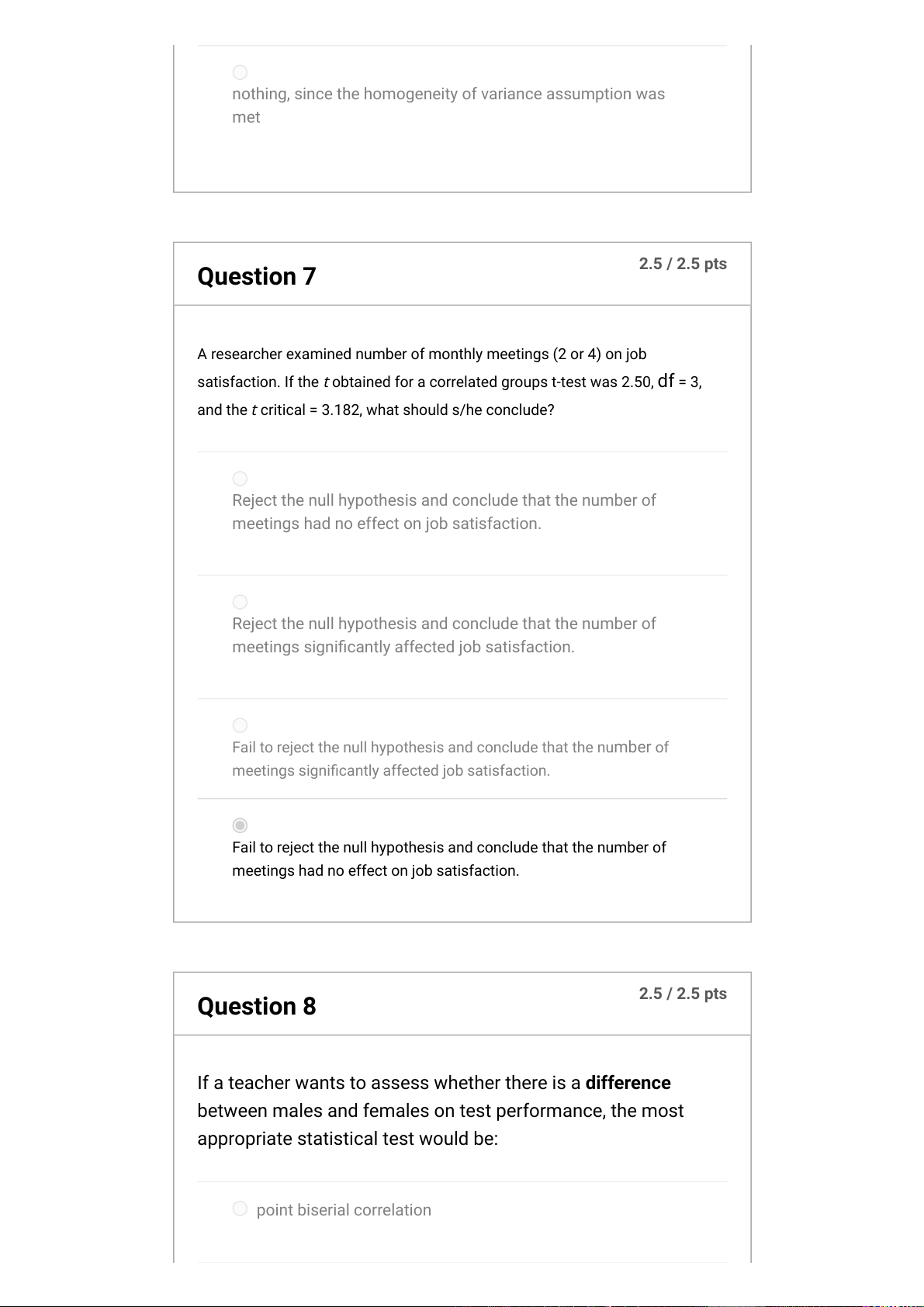nothing, since the homogeneity of variance assumption was
met
2.5 / 2.5 pts
Question 7
A researcher examined number of monthly meetings (2 or 4) on job
satisfaction. If the
t
obtained for a correlated groups t-test was 2.50,df= 3,
and the
t
critical = 3.182,what should s/he conclude?
Reject the null hypothesis and conclude that the number of
meetings had no effect on job satisfaction.
Reject the null hypothesis and conclude that the number of
meetings signicantly affected job satisfaction.
Fail to reject the null hypothesis and conclude that the numberof
meetings signicantly affected job satisfaction.
Fail to reject the null hypothesis and conclude that the number of
meetings had no effect on job satisfaction.
2.5 / 2.5 pts
Question 8
If a teacher wants to assess whether there is a difference
between males and females on test performance, the most
appropriate statistical test would be:
point biserial correlation